Here is a correct way of defining a function:

> f:= x -> x^2 + 1;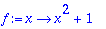You can then evaluate such a function with a constant or variable argument:

> f(u);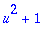> f(3);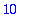On the other hand, the following defines an expression rather than a function:

> g:= x^2 + 1;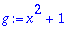This is no problem if that's what you wanted, but functions and expressions are used in different ways. In particular, you get a strange-looking result if you try to evaluate g as you would a function:

> g(u);In order to evaluate g for some value of x , you must use subs .

> subs(x=u, g);Since in ordinary mathematics we often speak of "the function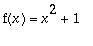", a common beginner's mistake is to define f(x) instead of defining f .

> h(x):= x^2 + 1;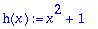Beware! Everything seems fine, as long as you use only h(x) . But when you try to use h with some other argument, it appears to be undefined:

> h(u);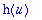This is not surprising, since in fact it is only h(x) , and not h of anything else, that you have defined.

There is a legitimate use of such a construction (it places a value in the remember table of h ).